# Jordan matrix

also Jordan canonical form, Jordan normal form

A square block-diagonal matrixover a fieldof the form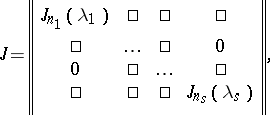where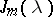is a square matrix of orderof the form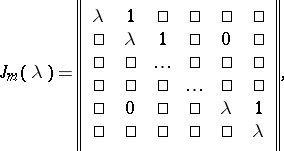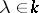. The matrixis called the Jordan block of orderwith eigen value. Every block is defined by an elementary divisor (cf. Elementary divisors, see ).

For an arbitrary square matrixover an algebraically closed fieldthere always exists a square non-singular matrixoversuch that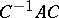is a Jordan matrix (in other words,is similar overto a Jordan matrix). This assertion is valid under weaker restrictions on: For a matrixto be similar to a Jordan matrix it is necessary and sufficient thatcontains all roots of the minimum polynomial of. The matrixmentioned above is called a Jordan form (or Jordan normal form) of the matrix. C. Jordan  was one of the first to consider such a normal form (see also the historical survey in Chapts. VI and VII of ).

The Jordan form of a matrix is not uniquely determined, but only up to the order of the Jordan blocks. More exactly, two Jordan matrices are similar overif and only if they consist of the same Jordan blocks and differ only in the distribution of the blocks along the main diagonal. The number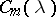of Jordan blocks of orderwith eigen valuein a Jordan form of a matrixis given by the formula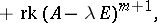whereis the unit matrix of the same orderas,is the rank of the matrix, and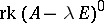is, by definition.

There are other types of normal forms of matrices besides a Jordan normal form. They are resorted to, for example, when it is desired to avoid the non-uniqueness of the reduction to a Jordan normal form, or when the ground field does not contain all roots of the minimum polynomial of the matrix (see ).

From the point of view of the theory of invariants, a Jordan matrix is a canonical representative in the orbits of the adjoint representation of the general linear group. The determination of analogous representatives for an arbitrary reductive algebraic group is still (1978) not completely solved (see ).